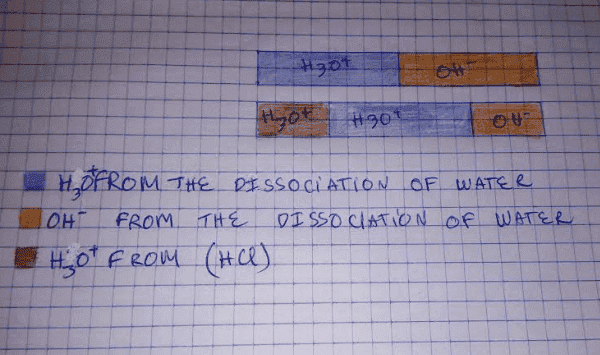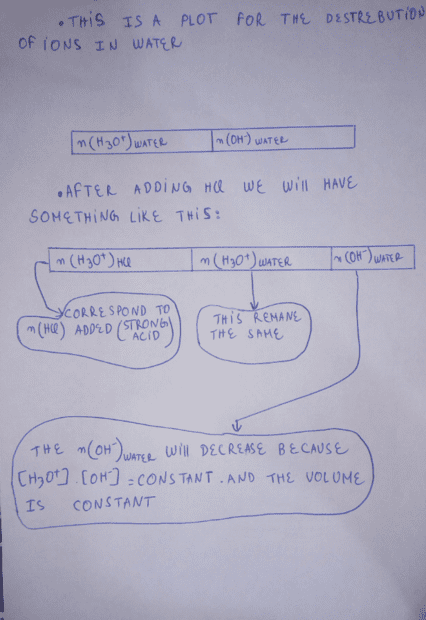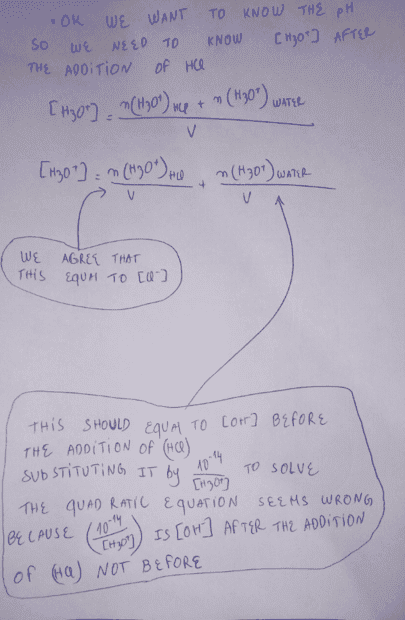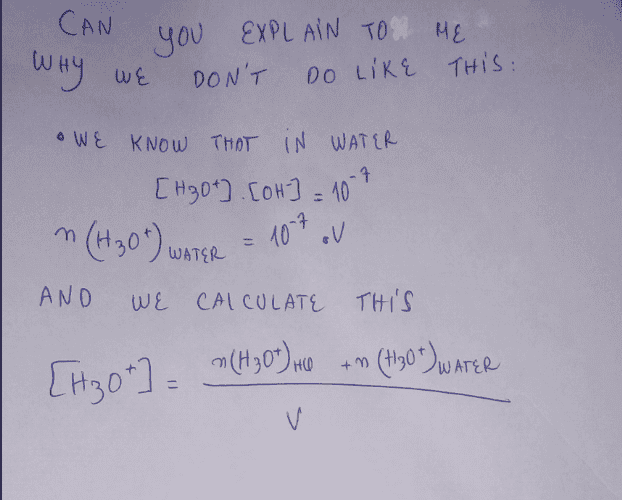# Something that doesn't make sense to me about calculating the pH

• samy4408
H3O+] in the solution will change and it will reach a new equilibrium In summary, In a lecture entitled "calculating the pH of a strong acid/base solution ", after adding a certain amount of HCl in water, we are asked to calculate the pH. We use Kw to replace [OH-] with (10^-14/[H3O+]) and solve for [H3O+] using the quadratic equation. However, when we add HCl to a solution containing a salt of a weak base, the concentration of [H3O+] does not always equal [Cl^-], and the quadratic equation is unnecessary.f

#### samy4408

Hello , the problem that i refer to is present when we try to calculate the pH of a solution without any assumption.

in a lecture entitled "calculating the pH of a strong acid/base solution " after adding a certain amount of HCl in water we are asked to calculate the pH
(the amount of HCl and volume of water is known )

*to calculate the pH we need to calculate [H3O+]
*we have 2 sources of [H3O+] in this solution
-first the [H3O+] from HCl
-second the [H3O+] from the dessosiation of water

we know that [H3O+]from HCl=[Cl-] because HCl is a strong acid
and we reffered to the [H3O+] from water as [OH-] ( here is the problem but we'll come back to it later )

using Kw we replaced [OH-] with (10^-14/[H3O+])
now we have this equation [H3O+]=(10^-14/[H3O+]) +[Cl-]
and the professor said that all we need to do is to solve the quadratic equation

here is the problem it seem that the two [H3O+] are not the same unknown ( the amount of [H3O+] from water do not equal to (10^-14/[H3O+])) , here is a plotMaybe this not deep enough for you but if just HCl in water, then the important amount of hydronium ion concentration comes from the HCl. One usually wants to account for other hydronium ion source when the solution contains a salt of a weak base in the solution; and then the use of a quadratic equation becomes more significant.

@Borek could explain this much more deeply.

There is no such thing as two different H3O+ concentrations in the solution. All H3O+ look the same and participate in the same reactions, there is no way to say where do they come from, so in every equilibrium and every mass/charge balance only the total concentration matters.

There are specific cases where expressing the total as a sum of concentrations coming from different sources is a neat trick helping in calculations, but this one here is not one of them.

The dissociation of water is usually so insignificant that it is neglected, with the exception of very dilute solutions.

The solution that the OP @samy4408 presents sets the positive ion concentration equal to the negative ion concentration to balance the charge. The result with a little algebra is a quadratic expression for the ## [H^+ ] ##. You can then solve for ## [OH^-] =10^{-14}/[H^+]##, and oftentimes it will be so much less than the ## [Cl^-] ##, that it can be ignored, and the quadratic solution is unnecessary. In that case, ## [H^+] \approx [Cl^-] ##, (where we began with ## [H^+]=[OH^-]+[Cl^-] ##).

Last edited:
@samy4408 -- Please learn to use LaTeX to post math equations, and type your work into the forum window. Your images in the post above are basically illegible to me. The "LaTeX Guide" below the Edit window is a good place to start. Thank you.

There is no such thing as two different H3O+ concentrations in the solution. All H3O+ look the same and participate in the same reactions, there is no way to say where do they come from, so in every equilibrium and every mass/charge balance only the total concentration matters.

There are specific cases where expressing the total as a sum of concentrations coming from different sources is a neat trick helping in calculations, but this one here is not one of them.
Hello , i understood my misconception about the concentration , the following is a reformulation of my problem : sorry i tried to use latex but i found it less comprehensible than thisYou don't even need LaTeX. If what you see on your screen is the same as on mine at the top of the posting form symbols for getting subscripts and superscripts, on far right a button for seeing what the result will be - all you need here.

As far as I can make out you are still thinking about two separate H3O+ types that belong to themselves separately.

Also you are only using two (the equilibrium constant expressions) of the three equations you would need to solve the problem completely exactly. The one you are missing is the one that expresses electroneutrality of the whole solution.

Write out also the formula for that and you may find that you are led to a correct solution and way of looking at it.

hello , thanks for your replay .
finally i found the answer for my problem , it's just about le Chatelier's principle when we add HCl , [OH-] decreases by reacting with H3O+ to form H2O
so H3O+ native to water also decreases , then it makes sens to solve the quadratic equation .

•Yes, that's a direct result of what I wrote earlier - there are no "different" ions of the same kind - all H+ is involved in every reaction.

There are some other problems with what you wrote, but they are impossible to quote. For example, you wrote "we agree (this concentration of H+) equal [Cl-" - but we don't, for the same reason. There is no such thing as "this concentration". If anything, we can agree that

$$[H^+] = [Cl^-] + [OH^-]$$

which just means solution is electrically neutral (this is so called "charge balance equation").

If I start with the recognition that pH means 'potential Hydrogen', then what @Borek said might be for me conceptually even more well-anchored ##-##1. pH related questions are about the most common in homework help. I usually answer that there are just these four things you have to use:

i) The defining equations for equilibrium constants

ii) equations for conservation of mass (maybe better called 'conservation of molarity')

iii) conservation of charge, or electroneutrality equation

iv) relevant approximations.

2a) I've noticed that students have usually got the first two, and forgetting (iii) is what is stopping them solving the problem.

2b) an odd thing, whenever I asked a student 'write the electroneutrality condition' the threaded ended there, I think without exception, there was never any reply. I don't know whether this is because it makes it all seem easy, or it is too difficult.

3) The approximations, and understanding their extent and limits, are not just an add-on convenience, but an essential part of what needs to be grasped when you learn this subject. Subsequently it becomes intuitive, helps prevent error, there are endless occasions to apply it, and it is all part of the routine thinking of laboratory biochemists etc.

4
it's just about le Chatelier's principle when we add HCl , [OH-] decreases by reacting with H3O+ to form H2O
so H3O+ native to water also decreases , then it makes sense to solve the quadratic equation .
In this case, for example if HCl is 0.1M, then to excellent approximation [H+] = [Cl-] = 0.1. That is all that is needed for all practical purposes. But here we are interested in tying up our theoretical understanding - and then I could say that since by the equilibrium equation [OH-] = 10-13, according to the electroneutrality condition

[H+] = 0.1 + 10-13

an extremely minuscule correction. I have not needed to solve a quadratic. (It may be the first time I have thought about it at all! - though I have come across quadratics in related problems in this area). You can, as you say solve the quadratic but I think it will not give you any better answer. In fact it may not give you an answer at all if you just depend on the routine formula! And then my correction is itself dependent on an approximation. I could further correct it but it would be going to a ridiculous degree of precision.

5 Sometimes the perspective of students is so dominated by having to calculate [H3O+] that it seems the most important, the biggest thing. It is and it isn't. A small quantity that tells about the state of the big players in a solution, like, say, the balance, in a phosphate buffer between the far more abundant H2PO4- and HPO42-.

And then the volume of E. coli cytoplasm is about 7×10-16 - at its slightly above neutral pH it will contain averagely about 1 molecule of H3O+! But we wouldn't say its pH, a macroscopic concept, is fluctuating infinitely! The average protonation of a more abundant component e.g. side chains of ionizable amino acids, will vary less around their bulk average value

Last edited:
•2c) this 'charge conservation' is not really some separate scientific principle, is just a form of mass conservation, but It is helpful to think of it separately as the 'electroneutrality' idea makes the equation (in this case Borek's in #10) very easy to arrive at, I find.

Charge and mass are fundamentally different, while the math is very similar I wouldn't one call just "the variant of the other".

Quadratic equation becomes a must for diluted solutions, at some point if you ignore water autodissociation you are getting pH over 7 for a diluted acid solution, which is an obvious nonsense. Yes, such diluted solutions of strong acids have no much practical importance.

•